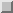## Examples of the FORALL Statement

FORALL (j=1:m, k=1:n) x(k,j) = y(j,k) FORALL (k=1:n) x(k,1:m) = y(1:m,k) These statements both copy columns 1 throughof arrayinto rows 1 throughof array. This is equivalent to the standard Fortran 90 statement

x(1:n,1:m) = TRANSPOSE(y(1:m,1:n)) FORALL (i=1:n, j=1:n) x(i,j) = 1.0 / REAL(i+j-1) This FORALL sets array elementto the valuefor values ofandbetween 1 and. In Fortran 90, the same operation can be performed by the statement

x(1:n,1:n) = 1.0/REAL( SPREAD((/(i,i=1,n)/),DIM=2,NCOPIES=n) & + SPREAD((/(j,j=1,n)/),DIM=1,NCOPIES=n) - 1 ) Note that the FORALL statement does not imply the creation of temporary arrays and is much more readable.

FORALL (i=1:n, j=1:n, y(i,j).NE.0.0) x(i,j) = 1.0 / y(i,j) This statement takes the reciprocal of each nonzero element of arrayand assigns it to the corresponding element of array. Elements ofthat are zero do not have their reciprocal taken, and no assignments are made to the corresponding elements of. This is equivalent to the standard Fortran 90 statement

WHERE (y(1:n,1:n) .NE. 0.0) x(1:n,1:n) = 1 / y(1:n,1:n) TYPE monarch INTEGER, POINTER :: p END TYPE monarch TYPE(monarch) :: a(n) INTEGER, TARGET :: b(n)

! Set up a butterfly pattern FORALL (j=1:n) a(j)This FORALL statement sets the elements of arrayto point to a permutation of the elements of. Whenand, then elements 1 through 8 ofpoint to elements 3, 4, 1, 2, 7, 8, 5, and 6 of, respectively. This requires a DO loop or other control flow in Fortran 90.

FORALL ( i=1:n ) x(indx(i)) = x(i) This FORALL statement is equivalent to the Fortran 90 array assignment

x(indx(1:n)) = x(1:n) Ifcontains a permutation of the integers from 1 to, then the final contents ofwill be a permutation of the original values. Ifcontains repeated values, neither the behavior of the FORALL nor the array assignment are defined by their respective standards.

FORALL (i=2:4) x(i) = x(i-1) + x(i) + x(i+1) If this statement is executed withthen after execution the new values of arraywill beThis has the same effect as the Fortran 90 statement

x(2:4) = x(1:3) + x(2:4) + x(3:5) Note that it does not have the same effect as the Fortran 90 loop

DO i = 2, 4 x(i) = x(i-1) + x(i) + x(i+1) END DO FORALL (i=1:n) a(i,i) = x(i) This FORALL statement sets the elements of the main diagonal of matrixto the elements of vector. This cannot be done by an array assignment in Fortran 90 unless EQUIVALENCE or WHERE is also used.

FORALL (i=1:4) a(i,ix(i)) = x(i) This FORALL statement sets one element in each row of matrixto an element of vector. The particular elements inare chosen by the integer vector. Ifand arrayrepresents the matrixbefore execution of the FORALL, thenwill representafter its execution. This operation cannot be accomplished with a single array assignment in Fortran 90.

FORALL (k=1:9) x(k) = SUM(x(1:10:k)) This FORALL statement computes nine sums of subarrays of x. (SUM is allowed in a FORALL because Fortran 90 intrinsic functions are pure; see Section.) If before the FORALLthen after the FORALLThis computation cannot be done by Fortran 90 array expressions alone.Next:
Scalarization of the Up: The FORALL Statement Previous: Interpretation of Element

paula@erc.msstate.edu
Thu Jul 21 17:05:43 CDT 1994